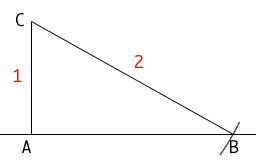Hi! I am a Math 7/8 teacher. I was wondering how you would show a student how to find the exact location of the square root of three on the number line using just a compass and a straight edge. Thanks! Sincerely, Allan Hi Allen, One way you can do this is to use the Theorem of Pythagoras. Draw a line. At a point A on the line construct a line which is perpendicular to the first line. Mark a point C on this line a distance of one unit from A.Using the compass, draw an arc of a circle with radius 2 and center C so that the arc intersects the first line at B. Draw the line segment CB. Now you have a right triangle with hypotenuse of length 2 units and one side of length 1 unit. Hence, by Pythagoras' Theorem |CA| 2 + |AB| 2 = |BC| 2 That is 1 + |AB| 2 = 4 and thus |AB| 2 = 3 and |AB| is the square root of 3. Cheers, Peny Go to Math Central